Browse by Topic
Related Topics

# The ABCD of Divergent Series

What is the sum of all the natural numbers? Intuition suggests that the answer is infinity, and, in calculus, the natural numbers provide a simple example of a divergent series. Yet mathematicians and physicists have found it useful to assign fractional, negative, or even zero values to the sums of such series. My aim in writing this post is to clear up some of the mystery that surrounds these seemingly bizarre results for divergent series. More specifically, I will use Sum and other functions in Mathematica to explain the sense in which the following statements are true.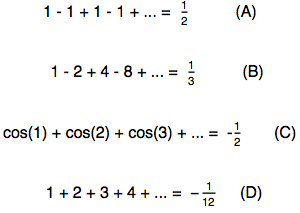The significance of the labels A, B, C, and D for these examples will soon become clear!

To begin with, let us recall the notion of a convergent infinite series using the following geometric series.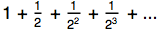The general term of the series, starting at n = 0, is given by: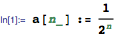We now define the sum of the series from i = 0 up to some finite value i = n.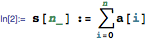This finite sum is called a partial sum for the series.

A plot of the partial sums shows that they approach 2 as the value of n increases: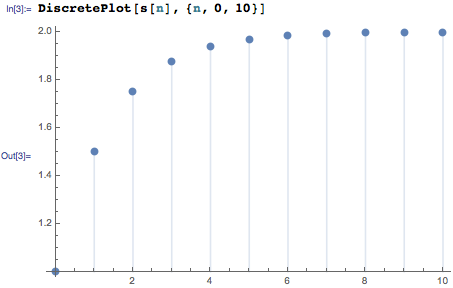Limit confirms that the partial sums approach 2 as n approaches infinity.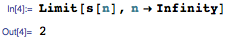Sum gives the same result for the sum from 0 to infinity.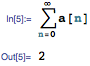We say that the above geometric series is convergent and that its sum is 2.

In general, an infinite series is convergent if the sequence of its partial sums approaches a definite value as the number of terms approaches infinity. In this case, the limiting value of the partial sums is called the sum of the series.

An infinite series that is not convergent is said to be divergent. By definition, divergent series cannot be summed using the method of partial sums that we illustrated above. However, mathematicians have devised various means of assigning finite values to such series. Such a finite value is called a regularized sum for the divergent series. The process of computing regularized sums is called regularization or resummation.

We are now ready to consider example A from the introduction.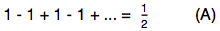The label A stands for Abel (Niels Henrik Abel, 1802–1829), the famous Norwegian mathematician who provided a technique for regularizing a divergent series. During his brief life of 26 years, Abel made spectacular progress with solving some of the hardest mathematical problems. In particular, he showed that the general algebraic equation of fifth degree could not be solved in radicals, a problem that had remained unsolved for 250 years before his time.

In order to apply Abel’s method, we note that the general term of the given series is: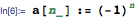This can be seen by making a table of the first few values for a[n].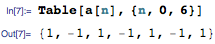As the following plot shows, the partial sums have values 1 or 0 depending on whether n is even or odd.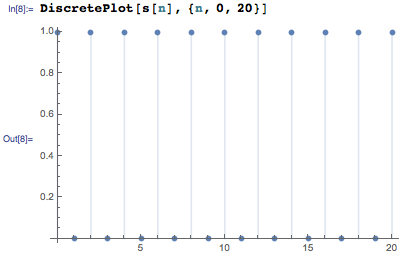Hence, Sum issues a message to indicate that the series is divergent.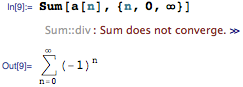Abel regularization can be applied to this series in two steps. During the first step, we sum the corresponding power series.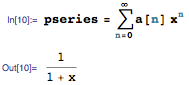Next we take the limit of this sum as x approaches 1, noting that the power series converges for values of x arbitrarily close to, but less than, 1.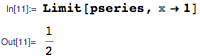These two steps can be combined to define the Abel sum of the series.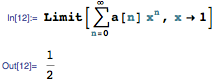We can obtain the same answer using the Regularization option for Sum as follows.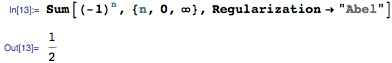The answer 1/2 seems reasonable, since it is the average of the two possible values, 1 and 0, for the partial sums of this series. Also, the limiting procedure by which it was calculated is very intuitive because with x = 1, the power series coincides with the given infinite series. However, Abel was greatly concerned about the lack of rigor that existed in mathematical analysis before his time, and cautioned that:

“Divergent series are the invention of the devil, and it is shameful to base on them any demonstration whatsoever. By using them, one may draw any conclusion he pleases and that is why these series have produced so many fallacies and so many paradoxes.” (N. H. Abel in a letter to his former teacher Berndt Holmböe, January 1826)

We now turn to example B, which states that: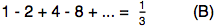The label B stands for Borel (Émile Borel, 1871–1956), a French mathematician who worked on topics such as measure theory and probability. In particular, Borel is associated with the infinite monkey theorem, which states that a monkey hitting a random sequence of keys on a typewriter keyboard for an infinite amount of time will almost surely type a given text, such as the complete works of William Shakespeare.

In order to apply Borel’s method, we note that the general term of the given series is: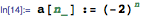Borel regularization can be applied to this rapidly growing divergent series in two steps. During the first step, we calculate the exponential generating function of the series. The n! in the denominator below ensures that the infinite series converges for all values of t.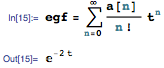Next, we compute the Laplace transform of the exponential generating function at s = 1.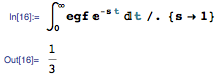These two steps can be combined to define the Borel sum of the series.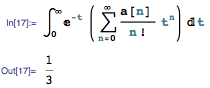The answer can be obtained directly using Sum as follows.The definition of the Borel sum makes sense because it gives the same result as that obtained using the method of partial sums when it is applied to convergent series. For, in this case, one can interchange summation and integration, and then the definition of the Gamma function gives: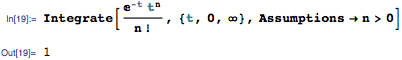However, such an interchange is not valid for divergent series, which leads to interesting results when Borel regularization is applied to such series.

Borel summation provides a versatile technique for evaluating divergent series that occur in quantum field theory, and there is much literature on this topic.

Example C on our list states that: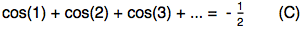The label C stands for Cesàro (Ernesto Cesàro,1859–1906), an Italian mathematician who made significant contributions to differential geometry, number theory, and mathematical physics. Cesàro was a prolific mathematician and wrote around 80 works during the period 1884–86, before being awarded a PhD in 1887!

As a first step in applying Cesàro’s method, we note that the general term of the series, starting at n = 0, is: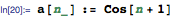A plot of the partial sums shows that they oscillate wildly.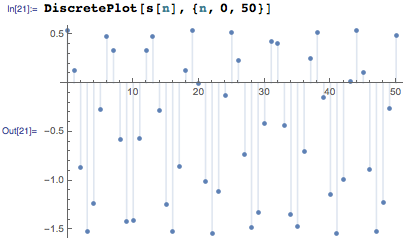Cesàro’s method essentially uses successive averages of the partial sums to tame these oscillations, as seen in the following plot.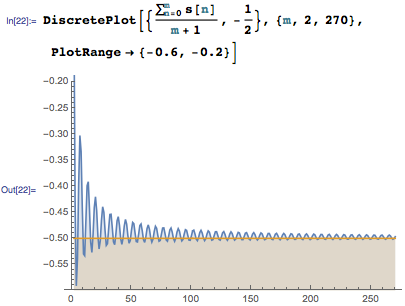Formally, the Cesàro sum is defined as the limit of the successive averages of the partial sums. Computing this limit gives the expected answer -1/2 for example C.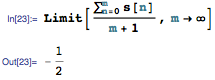The Cesàro sum can be obtained directly by setting Regularization -> "Cesaro" in Sum, as shown below.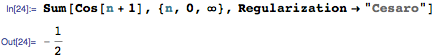Cesàro sums play an important role in the theory of Fourier series, which are trigonometric series used to represent periodic functions. The Fourier series for a continuous function may not converge, but the corresponding Cesàro sum (or Cesàro mean, as it is usually called) will always converge to the function. This beautiful result is called Fejér’s theorem.

Our final example requires us to show that the sum of the natural numbers is -1/12.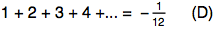The label D stands for Dirichlet (Johann Peter Gustav Lejeune Dirichlet, 1805–1859), a German mathematician who made deep contributions to number theory and several other areas of mathematics. The breadth of Dirichlet’s contributions to the subject can be gauged by simply typing in the following wildcard search in Mathematica 10.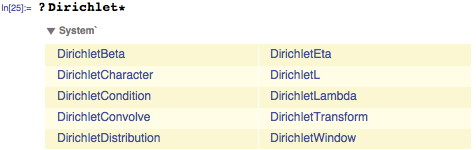Dirichlet’s method of regularization takes its name from the notion of a Dirichlet series, which is defined by: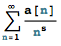A special case of this series defines the Riemann Zeta function.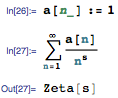SumConvergence informs us that this series converges only if the real part of s is greater than 1.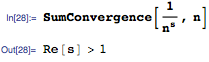However, the zeta function itself can be defined for other values of s using the process of analytic continuation from complex analysis. For example, with s = -1, we get: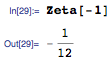But with s = -1, the series defining Zeta is precisely the series of natural numbers. Hence we get: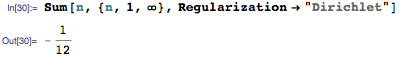Another way to understand this answer is to introduce a parameter ε into the infinite sum and then apply Series, as shown below.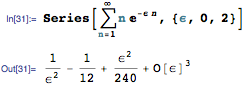The first term 1/ε2 in the above series expansion diverges as ε approaches 0, while the third term ε2/240 as well as all higher terms become infinitely small when taking this limit. If we discard these terms, then we are left with the Dirichlet sum -1/12 for the natural numbers. Thus, the Dirichlet sum is obtained by discarding both the infinitely small and the infinitely large terms in the series expansion. This is in sharp contrast to the convention of ignoring only infinitely small (infinitesimal) quantities that is used in college calculus, and accounts for the non-intuitive nature of the result given for the Dirichlet sum of the natural numbers.

The same argument can be used to justify the bizarre value 0 for the regularized sum of the squares of the natural numbers.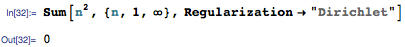In this case, we are left with 0 after discarding terms with positive or negative powers of ε in the series expansion.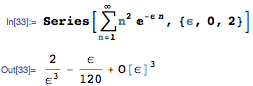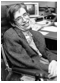Dirichlet regularization is closely related to the process of zeta regularization used in modern theoretical physics. In a celebrated paper, the eminent British physicist Stephen Hawking (1977) applied this technique to the problem of computing Feynmann path integrals in a curved spacetime. Hawking’s paper described the process of zeta regularization in a systematic manner to the physics community, and has been cited widely since it was published.

Our knowledge about divergent series is based on profound theories developed by some of the finest thinkers of the last few centuries. Yet, I sympathize with readers who, like me, feel uneasy about their use in current physical theories. The mighty Abel may have been right when he called these series the invention of the devil. And it is possible that a future Einstein, working in isolation, will cast aside prevailing dogma and reformulate fundamental physics to avoid the use of divergent series. But even if such developments do take place, divergent series will already have provided us with a rich source of mathematical ideas, and will have illumined the way to a deeper understanding of our universe.